Learning curves are a widely used diagnostic tool in machine learning for algorithms such as deep learning that learn incrementally. During training time, we evaluate model performance on both the training and hold-out validation dataset and we plot this performance for each training step (i.e. each epoch of a deep learning model or tree for an ensembled tree model). Reviewing learning curves of models during training can be used to diagnose problems with learning, such as an underfit or overfit model, as well as whether the training and validation datasets are suitably representative. In this notebook, I will illustrate to how you can use learning curves to:

1. Diagnose model behavior such as under or overfitting
2. Diagnose issues regarding disproportionate data representation

This notebook will demonstrate these issues with learning curve plots but does not show any code.1

## Diagnosing Model Behavior

The shape and dynamics of a learning curve can be used to diagnose the behavior of a machine learning model and in turn perhaps suggest at the type of configuration changes that may be made to improve learning and/or performance. There are three common dynamics that you are likely to observe in learning curves:

• Underfit
• Overfit
• Optimal Fit

We will take a closer look at each with examples. The examples will assume that we are looking at a minimizing loss metric, meaning that smaller relative scores on the y-axis indicate better performance.

### Underfit learning curves

Underfitting refers to a model that has not adequately learned the training dataset to obtain a sufficiently low training error value. There are two common signals for underfitting. First, our training learning curve may show a flat line or noisy values of relatively high loss, indicating that the model was unable to learn the training dataset at all. An example of this is provided below and is common when the model does not have a suitable capacity for the complexity of the dataset.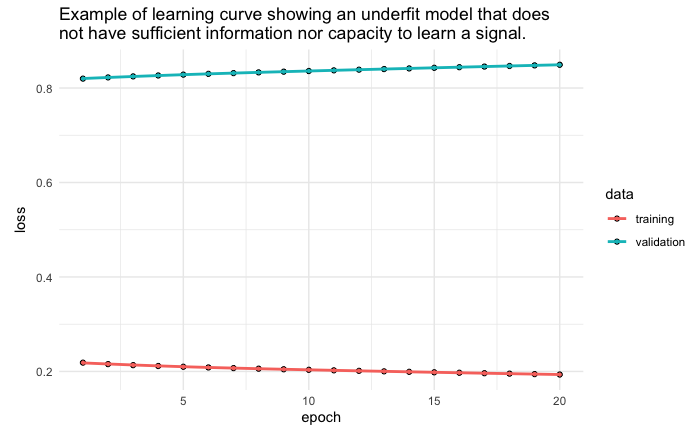Solution:

1. Add more observations. You may not have enough data for the existing patterns to become strong signals.
2. Add more features. Occasionally our model is under-fitting on the grounds that the feature items are insufficient.
3. Reduce any regularization on the model. If you have explicit regularization parameters specified (i.e. dropout, weight regularization), remove or reduce these parameters. ℹ️
4. Increase model capacity. Your model capacity may not be large enough to capture and learn existing signals. ℹ️

An underfit model may also be identified by a training and validation loss that are continuing to decrease at the end of the plot. This indicates that the model is capable of further learning and that the training process was halted prematurely.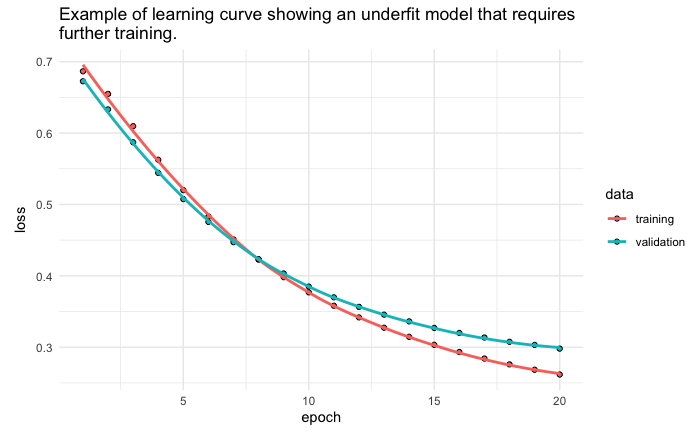Solution:

1. Increase the number of epochs until the validation curve has stopped improving. This is a good time to crank up the epochs and add an early stopping callback to identify how many epochs are required. ℹ️
2. If it is taking a long time to reach a minimum for the validation curve, increase the learning rate to speed up the gradient traversal and also add a callback to automatically adjust the learning rate. ℹ️

### Overfit learning curves

Overfitting refers to a model that has learned the training dataset too well, including the statistical noise or random fluctuations in the training dataset.

“… fitting a more flexible model requires estimating a greater number of parameters. These more complex models can lead to a phenomenon known as overfitting the data, which essentially means they follow the errors, or noise, too closely.”2

The problem with overfitting, is that the more specialized the model becomes to training data, the less well it is able to generalize to new data, resulting in an increase in generalization error. Overfitting is apparent when:

• the training loss continues to decrease with experience while
• the validation loss has decreased to a minimum and has begun to increase.

However, a model that overfits is not necessarily a bad thing. In fact, it signals that the model has extracted all the signal that that particular model could learn. The issues to be concerned about with overfitting is the magnitude and the inflection point.

A model that overfits early and has a sharp “U” shape often indicates overcapacity and/or a learning rate that is too high.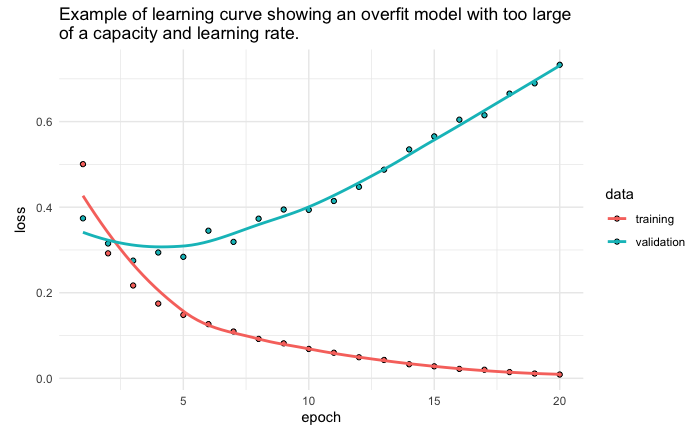Solution:

1. Regularize how quickly the model learns by reducing the learning rate. Add a callback to automatically reduce the learning rate as the validation loss plateaus. ℹ️
2. Regularize model capacity by reducing the number and/or size of the hidden layers. ℹ️
3. Regularize the weights to constrain the complexity of the network. ℹ️
4. Regularize happenstance patterns by adding dropout to minimize the chance of fitting patterns to noise in the data. ℹ️

Often, we can minimize overfitting but rarely can we completely eliminate it and still minimize our loss. The following illustrates an example where we have minimized overfitting, yet some overfitting still exists.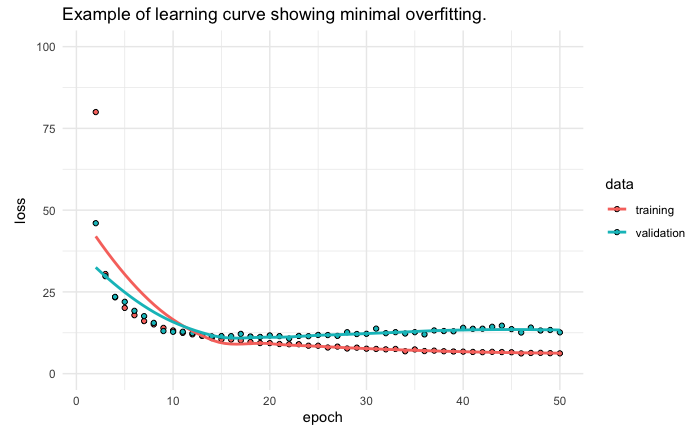Solution:

1. Add an early stopping callback to stop training once the validation curve has stopped improving. ℹ️
2. Add `restore_best_weights = TRUE` to your callback so that your final model uses the weights from the epoch with the best loss score.

### Optimal fit learning curves

An optimal fit is the goal of the learning algorithm. The loss of the model will almost always be lower on the training dataset than the validation dataset. This means that we should expect some gap between the train and validation loss learning curves. This gap is referred to as the generalization gap. An optimal fit is one where:

• The plot of training loss decreases to a point of stability.
• The plot of validation loss decreases to a point of stability.
• The generalization gap is minimal (nearly zero in an ideal situation).

Continued training of an optimal fit will likely lead to overfitting. The example plot below demonstrates a case of an optimal fit assuming we have found a global minimum of our loss function.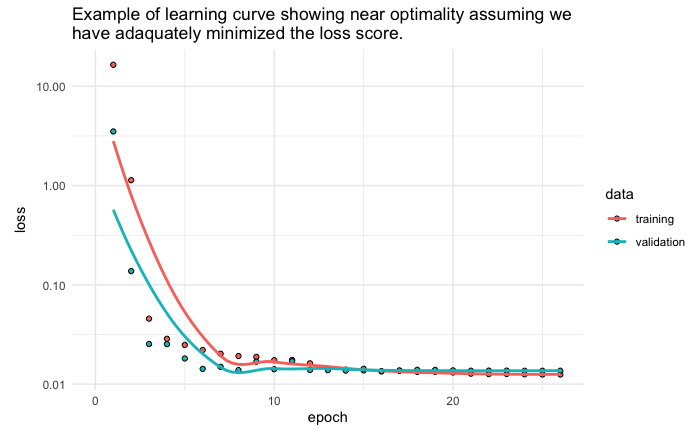## Diagnosing Unrepresentative Datasets

Learning curves can also be used to diagnose properties of a dataset and whether it is relatively representative. An unrepresentative dataset means a dataset that may not capture the statistical characteristics relative to another dataset drawn from the same domain, such as between a train and a validation dataset. This can commonly occur if the number of samples in a dataset is too small or if certain characteristics are not adequately represented, relative to another dataset.

There are two common cases that could be observed; they are:

• Training dataset is relatively unrepresentative
• Validation dataset is relatively unrepresentative

### Unrepresentative train dataset

An unrepresentative training dataset means that the training dataset does not provide sufficient information to learn the problem, relative to the validation dataset used to evaluate it. This situation can be identified by a learning curve for training loss that shows improvement and similarly a learning curve for validation loss that shows improvement, but a large gap remains between both curves. This can occur when

• The training dataset has too few examples as compared to the validation dataset.
• Training dataset contains features with less variance than the validation dataset.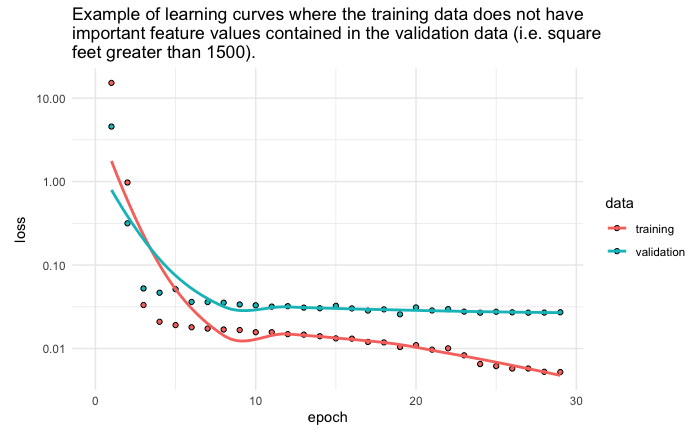Solution:

1. Add more observations. You may not have enough data to capture patterns present in both the training and validation data.
2. If using CNNs incorporate data augmentation to increase feature variability in the training data. ℹ️
3. Make sure that you are randomly sampling observations to use in your training and validation sets. If your data is ordered by some feature (i.e. neighborhood, class) then you validation data may have features not represented in your training data. ℹ️
4. Perform cross-validation so that all your data has the opportunity to be represented in both the training and validation sets. ℹ️

### Unrepresentative validation dataset

An unrepresentative validation dataset means that the validation dataset does not provide sufficient information to evaluate the ability of the model to generalize. This may occur if the validation dataset has too few examples as compared to the training dataset. This case can be identified by a learning curve for training loss that looks like a good fit (or other fits) and a learning curve for validation loss that shows noisy movements and little or no improvement.